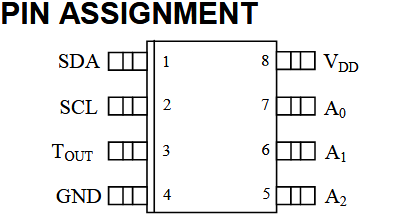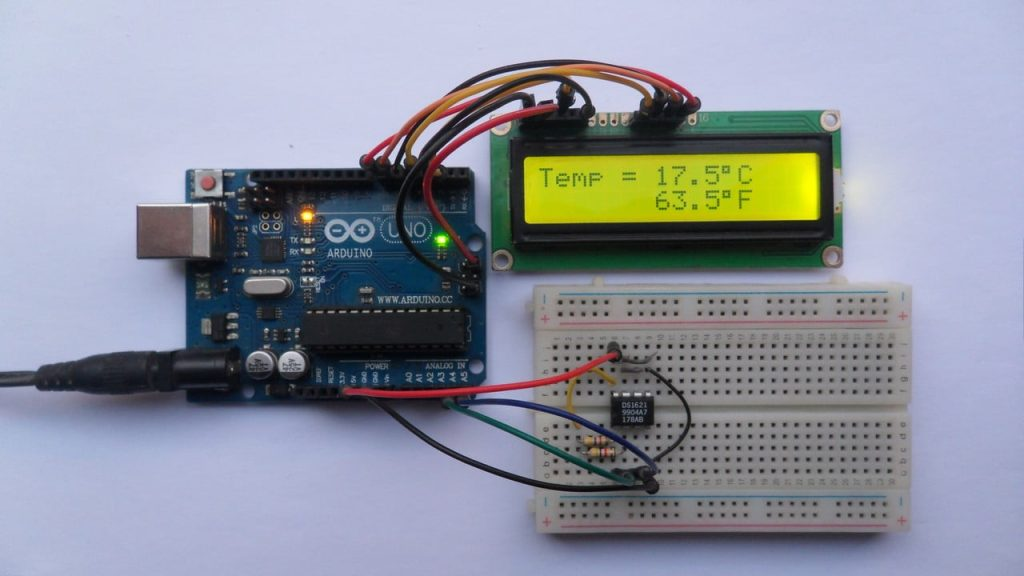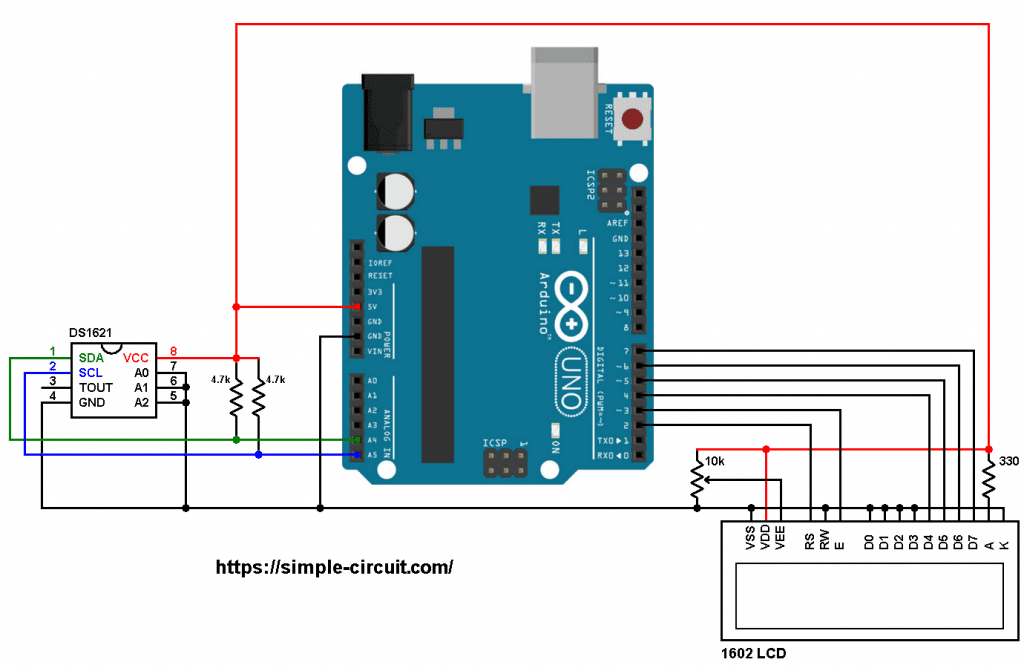# Interfacing Arduino with DS1621 Temperature Sensor

This post shows how to interface Arduino uno board with DS1621 digital temperature sensor where temperature values (in degrees Celsius and degrees Fahrenheit) are printed on 1602 LCD screen.

The DS1621 Digital Thermometer and Thermostat from Maxim Integrated provides 9-bit temperature readings, which indicate the temperature of the device. The thermal alarm output, TOUT, is active when the temperature of the device exceeds a user-defined temperature TH. The output remains active until the temperature drops below user defined temperature TL, allowing for any hysteresis necessary.

Benefits and features:

• Measures temperatures from – 55 °C to +125 °C in 0.5 °C increments. Fahrenheit equivalent is -67 °F to 257 °F in 0.9 °F increments.
• Temperature is read as a 9-Bit value (2-byte transfer).
• Converts temperature to digital word in less than 1 second.
• Thermostatic settings are user definable and nonvolatile.
• Power supply range (2.7V to 5.5V).
• Data is read from/written via a 2-wire serial interface (open drain I/O lines).
• Saves space.
• Temperature measurements require no external components.
• 8-pin DIP or SO package (208-mil) packages.Hardware Required:

• Arduino board
• 1602 LCD screen
• DS1621 temperature sensor   —->   datasheet
• 2 x 4.7k ohm resistor
• 330 ohm resistor
• 10k ohm variable resistor or potentiometer
• Jumper wiresInterfacing Arduino with DS1621 temperature sensor circuit:
Project circuit diagram is shown below.

The 1602 LCD screen (2 rows and 16 columns) is used to display temperature values in degrees Celsius and degrees Fahrenheit where:
RS —> Arduino digital pin 2
E   —> Arduino digital pin 3
D4 —> Arduino digital pin 4
D5 —> Arduino digital pin 5
D6 —> Arduino digital pin 6
D7 —> Arduino digital pin 7
VSS, RW, D0, D1, D2, D3 and K are connected to Arduino GND,
VEE to the 10k ohm variable resistor (or potentiometer) output,
VDD to Arduino 5V and A to Arduino 5V through 330 ohm resistor.

VEE pin is used to control the contrast of the LCD. A (anode) and K (cathode) are the back light LED pins.The DS1621 sensor SDA (serial data) and SCL (serial clock) pins are respectively connected to Arduino A4 and A5 pins (ATmega328P hardware I2C module pins). It is is supplied with 5V from the Arduino board.
The three pins: A0, A1 and A2 are slave address select pins which are connected to ground, therefore the DS1621 takes an address of 0x48 (1001+A2+A1+A0).

Arduino with DS1621 sensor and 16×2 LCD code:
Hints:
Since the address pins of the DS1621 (A2, A1 and A0) are connected to Arduino GND its I2C slave address is 0x48, it’s defined in the code as shown below:

A function named get_temperature() in the Arduino code is used to read raw temperature values from the DS1621 sensor and convert it to tenths degrees Celsius (output value of “135” equals 13.5 °Celsius).
This functions returns a signed integer number (2 bytes).

The temperature in tenths degrees Fahrenheit =  (tenth °Celsius) x 9/5 +320 (because: °F = °Cx9/5 + 32).

To get the actual value of each quantity we’ve to divide it by 10. The line below shows an example for temperature in °C:

We get the first 2 digits by dividing the tenths value by 10, and the tenths number (number after the decimal point) of the actual temperature value is equal to the reminder of that division (tenths value % 10).

The resolution of this thermometer is 0.5°C.

Full Arduino code:

Arduino with DS1621 sensor and 16×2 LCD Proteus simulation:
We can easily simulate this project with Proteus ISIS software. The following video shows how does it work: